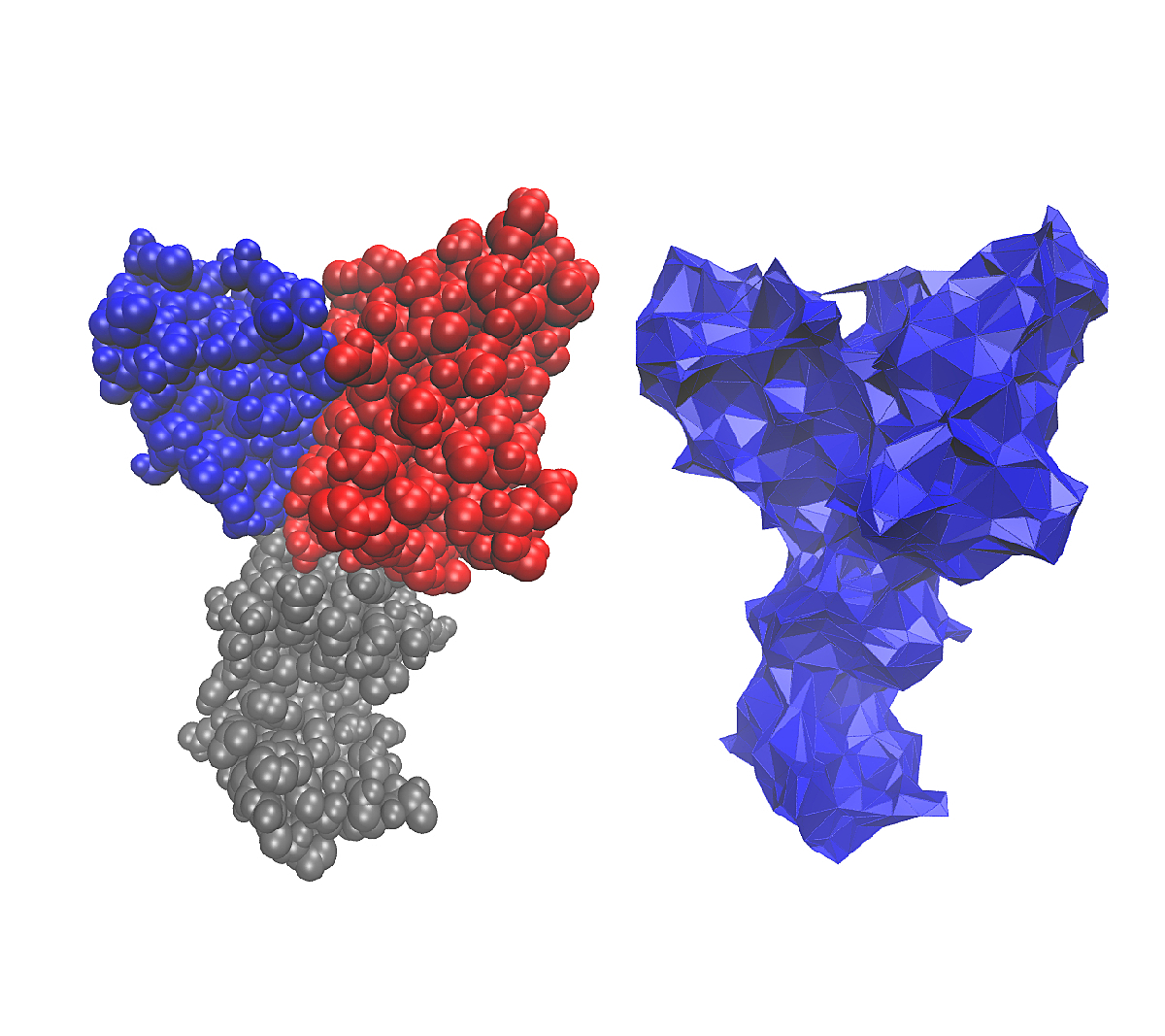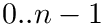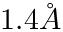Structural Bioinformatics Library Template C++ / Python API for developping structural bioinformatics applications.
User Manual# Alpha_complex_of_molecular_model

Authors: F. Cazals and T. Dreyfus

# Introduction

As explained in section Molecular structure, a molecular structure has two components:

• the molecular systems provide a hierarchy of groups of the particles of the molecular structure,
• the geometric model provides geometric features for each particle of the molecular structure, such as a shape and coordinates.

When particles are represented by 3D balls, a natural way to model the shape of a molecule is the 3D weighted-complex : it is a simplicial complex whose topology is exactly the one of the union of the particles.

This package provides wrappers for using the CGAL 3D-complex data structures over a collection of particles.

(Advanced) The-complex requires initial atomic radii for the input atoms. The choice of radii may impact the status of simplices, namely exterior, singular, regular, or interior. The case of 0-simplices i.e. vertices is of special interest. Indeed, depending on the atomic radii used, vertices corresponding to hydrogen atoms may be hidden or exterior. The first case corresponds to a hydrogen atom which does not have a Voronoi cell in the power diagram. The second one corresponds to a hydrogen atom which has a cell, yet, such that the restriction of this ball is empty. Such cases are rarely found and should trigger a careful inspection of the atomic coordinates.

# Implementation

This package was designed with a two-fold aim:

• being as most as possible generic: the-complex of particles data structure can be highly customized; in particular, the CGAL library defines two different data structures for representing the-complex: both can be used for representing the-complex of particles;
• being as most as possible simple: except the representation of a particle, all template parameters have a default type.

In the following, we introduce the main classes, then the different functionality of the package Alpha_complex_of_molecular_model .

## Main Classes

The main class is SBL::CSB::T_Alpha_complex_of_molecular_model< ParticleTraits, TAlphaComplexVertexBase , TAlphaComplexCellBase , TAlphaComplexBase > . It inherits from the template parameter TAlphaComplexBase and adds the functionality for inserting a particle whose type is provided by the concept ParticleTraits. The four template parameters are:

• ParticleTraits : concept that defines the type of the particle (Particle_type), the geometric primitives to use (Geometric_kernel) and the geometric representation of the particle (Get_geometric_representation).
• TAlphaComplexBase : base representation of the-complex templated by its underlying triangulation and a tag checking if critical values ofare exactly compared during the algorithm computing the-complex. Two models exist: (i) the CGAL class CGAL::Alpha_shape_3, or (ii) the SBL class SBL::CSB::T_Alpha_complex_base_3 that directly inherits from the CGAL class CGAL::Fixed_alpha_shape_3. While (i) allows to dynamically change the-value, (ii) is faster. The default type is (ii).
• TAlphaComplexVertexBase : base representation of a vertex in the underlying triangulation of the-complex. It is templated itself such that tow models can be used: (i) the CGAL class CGAL::Alpha_shape_vertex_base_3 or (ii) the SBL class SBL::CSB::T_Alpha_complex_vertex_base_3 that directly inherits from the CGAL class CGAL::Fixed_alpha_shape_vertex_base_3. The default type is (ii).
• TAlphaComplexCellBase : base representation of a cell in the underlying triangulation of the-complex. It is templated itself such that tow models can be used: (i) the CGAL class CGAL::Alpha_shape_cell_base_3 or (ii) the SBL class SBL::CSB::T_Alpha_complex_cell_base_3 that directly inherits from the CGAL class CGAL::Fixed_alpha_shape_cell_base_3. The default type is (ii).

The type of the underlying triangulation is the class SBL::CSB::T_Triangulation_of_molecular_model< ParticleTraits, TAlphaComplexVertexBase , TAlphaComplexCellBase >, whose template parameters were already described.

Finally, types such as geometric and combinatorial primitives, and the base data structure of the 3D triangulation are automatically instantiated from the traits class SBL::CSB::T_Alpha_complex_of_molecular_model_traits< ParticleTraits, TAlphaComplexVertexBase , TAlphaComplexCellBase >, where the template parameters are the same as the class SBL::CSB::T_Triangulation_of_molecular_model.

Three examples are given for illustrating the different possibilities of use of the class SBL::CSB::T_Alpha_complex_of_molecular_model :

• a case using the CGAL class CGAL::Alpha_shape_3 is shown in section Varying Alpha .

## Serialization

Vertices of the CGAL-complex data structures are partially serialized from the package Alpha_complexes_extensions. In addition, the base class SBL::CSB::T_Particle_vertex_base, used for representing vertices in the-complex with an attached particle, is serialized. In order to use the serialization of the vertices, one just has to include the file "SBL/IO/Alpha_complex_of_molecular_model_serialize.hpp" in its own source file.

## Molecular Viewers

Vertices, edges and faces of the-complex of particles are drawable by including the file "SBL/IO/Alpha_complex_of_molecular_model_molecular_draw.hpp", as explained in the package Molecular_viewers. An example of use is shown on in section Drawing the Simplices

## Module

This package provides also the module SBL::CSB:T_Alpha_complex_of_molecular_model_module< ModuleTraits > that computes the-complex of an input container of particles and computes statistics on the topology of the output-complex. The parameter ModuleTraits just has to define the input container type (Particles_container) and the output-complex type (Alpha_complex). An example of use is shown on in section Using the Module

This module requires that particles has a radius, that generally can be modified via options of the program. For example, default radii of atoms as defined in the package ParticleAnnotator are increased byto define the Solvent Accessible Model of the input molecule. This value can be modified with the option -r <value>. Note that this option differs from the option –alpha <value> of this module, which adds a fixed weight (homogeneous to a squared radius) to all balls during the computation of the-complex.

# Examples

## A Simple Example

In this example, atoms of a molecule are loaded from a PDB file, then annotated with default radii, and finally inserted in a-complex data structure. The number of finite simplices is printed at the end of calculations.

#include <SBL/CSB/Alpha_complex_of_molecular_model.hpp>
#include <SBL/Models/Atom_with_flat_info_and_annotations_traits.hpp>
#include <iostream>
typedef Alpha_complex::Triangulation Triangulation;
int main(int argc, char *argv[]){
if(argc < 2)
return -1;
//Loads a PDB file.
//Annotates the loaded atoms (default radii + 1.4 A from SAS model).
Particle_annotator annotator;
for(unsigned i = 0; i < atoms.size(); i++)
annotator(atoms[i]);
//Builds the alpha-complex of the loaded atoms.
Triangulation T(atoms.begin(), atoms.end());
//Number of simplices in the alpha-complex
unsigned nb_v = 0, nb_e = 0, nb_f = 0, nb_c = 0;
for(Alpha_complex::Finite_vertices_iterator it = A.finite_vertices_begin(); it != A.finite_vertices_end(); it++)
if(A.classify(it) != Alpha_complex::EXTERIOR) nb_v++;
for(Alpha_complex::Finite_edges_iterator it = A.finite_edges_begin() ; it != A.finite_edges_end() ; it++)
if(A.classify(*it) != Alpha_complex::EXTERIOR) nb_e++;
for(Alpha_complex::Finite_facets_iterator it = A.finite_facets_begin() ; it != A.finite_facets_end() ; it++)
if(A.classify(*it) != Alpha_complex::EXTERIOR) nb_f++;
for(Alpha_complex::Finite_cells_iterator it = A.finite_cells_begin() ; it != A.finite_cells_end() ; it++)
if(A.classify(it) != Alpha_complex::EXTERIOR) nb_c++;
std::cout << "#v, #e, #f, #c: " << nb_v << " " << nb_e << " " << nb_f << " " << nb_c << std::endl;
return 0;
}
Derived class from CGAL alpha-complex for filling it with particles.
Definition: Alpha_complex_of_molecular_model.hpp:598
Defines a generic serializable atom with annotations (default is name, radius and optional annotation...
Definition: Atom_with_flat_info_and_annotations_traits.hpp:121
Loader for one or more PDB files, even listed in a file. Loader for one or more PDB files,...
Collector for models of ParticleAnnotator.
Definition: Particle_annotator_collector.hpp:84
bool load(unsigned verbose, std::ostream &out)
Definition: Particle_annotator_collector.hpp:169
dictionary T
Definition: extract-wales-graph.py:35

## Varying Alpha

This example presents the CGAL class CGAL::Alpha_shape_3 with the SBL class SBL::CSB:T_Alpha_complex_of_molecular_model for varying the parameterafter constructing the-complex. This example is very similar to the example of section A Simple Example, except for three steps:

• the CGAL class CGAL::Alpha_shape_3 has a default mode called REGULARIZED such that singular simplices in the-complex are skipped: this mode is set to GENERAL in our case.
• if the user gives anvalue, thevalue of the-complex is changed and statistics are computed again.
#include <SBL/CSB/Alpha_complex_of_molecular_model.hpp>
#include <SBL/Models/Atom_with_flat_info_and_annotations_traits.hpp>
#include <iostream>
CGAL::Alpha_shape_vertex_base_3,
CGAL::Alpha_shape_cell_base_3,
CGAL::Alpha_shape_3> Alpha_complex;
typedef Alpha_complex::Triangulation Triangulation;
int main(int argc, char *argv[]){
if(argc < 2)
return -1;
//Loads a PDB file.
//Annotates the loaded atoms (default radii + 1.4 A from SAS model).
Particle_annotator annotator;
for(unsigned i = 0; i < atoms.size(); i++)
annotator(atoms[i]);
//Builds the alpha-complex of the loaded atoms (GENERAL mode is set
//to include all simplices, as opposed to the default REGULARIZED
//mode)
Triangulation T(atoms.begin(), atoms.end());
A.set_mode(Alpha_complex::GENERAL);
//Number of simplices in the alpha-complex
unsigned nb_v = 0, nb_e = 0, nb_f = 0, nb_c = 0;
for(Alpha_complex::Finite_vertices_iterator it = A.finite_vertices_begin(); it != A.finite_vertices_end(); it++)
if(A.classify(it) != Alpha_complex::EXTERIOR) nb_v++;
for(Alpha_complex::Finite_edges_iterator it = A.finite_edges_begin() ; it != A.finite_edges_end() ; it++)
if(A.classify(*it) != Alpha_complex::EXTERIOR) nb_e++;
for(Alpha_complex::Finite_facets_iterator it = A.finite_facets_begin() ; it != A.finite_facets_end() ; it++)
if(A.classify(*it) != Alpha_complex::EXTERIOR) nb_f++;
for(Alpha_complex::Finite_cells_iterator it = A.finite_cells_begin() ; it != A.finite_cells_end() ; it++)
if(A.classify(it) != Alpha_complex::EXTERIOR) nb_c++;
std::cout << "(alpha = 0) #v, #e, #f, #c: " << nb_v << " " << nb_e << " " << nb_f << " " << nb_c << std::endl;
if(argc < 3)
return 0;
//If an alpha value was given, change the alpha value of the
//alpha-complex and recompute the number of simplices in the
//alpha-complex.
double alpha = atof(argv);
A.set_alpha(alpha);
nb_v = 0, nb_e = 0, nb_f = 0, nb_c = 0;
for(Alpha_complex::Finite_vertices_iterator it = A.finite_vertices_begin(); it != A.finite_vertices_end(); it++)
if(A.classify(it) != Alpha_complex::EXTERIOR) nb_v++;
for(Alpha_complex::Finite_edges_iterator it = A.finite_edges_begin() ; it != A.finite_edges_end() ; it++)
if(A.classify(*it) != Alpha_complex::EXTERIOR) nb_e++;
for(Alpha_complex::Finite_facets_iterator it = A.finite_facets_begin() ; it != A.finite_facets_end() ; it++)
if(A.classify(*it) != Alpha_complex::EXTERIOR) nb_f++;
for(Alpha_complex::Finite_cells_iterator it = A.finite_cells_begin() ; it != A.finite_cells_end() ; it++)
if(A.classify(it) != Alpha_complex::EXTERIOR) nb_c++;
std::cout << "(alpha = " << alpha << ") #v, #e, #f, #c: " << nb_v << " " << nb_e << " " << nb_f << " " << nb_c << std::endl;
return 0;
}
alpha
Definition: gaussian_plot.py:48

## Running Union-Find over the Vertices

This example shows how to compute the-complex of a container of custom particle types. In particular, it adds a Union-Find structure to the particles and compute the number of connected component in the-complex.

#include <SBL/CSB/Alpha_complex_of_molecular_model.hpp>
#include <SBL/Models/Atom_with_flat_info_and_annotations_traits.hpp>
#include <iostream>
//Defines a particle type that is also a union-find vertex.
{
public:
};//end class Particle_traits
typedef Alpha_complex::Triangulation Triangulation;
int main(int argc, char *argv[]){
if(argc < 2)
return -1;
//Loads a PDB file.
//Annotates the loaded atoms (default radii + 1.4 A from SAS model).
Particle_annotator annotator;
std::list<Particle_traits::Particle_type> atoms;
for(Particle_traits::Molecular_system::Model::Atoms_iterator
it != loader.get_geometric_model().atoms_end(); it++)
{
atoms.push_back(Particle_traits::Particle_type());
atoms.back().build(*it);
annotator(atoms.back());
}
//Builds the alpha-complex of the loaded atoms.
Triangulation T(atoms.begin(), atoms.end());
//Computes the number of connected components in the model
Particle_traits::Union_find uf;
for(Alpha_complex::Finite_vertices_iterator it = A.finite_vertices_begin(); it != A.finite_vertices_end(); it++)
uf.make_set(&(*it).get_particle());
for(Alpha_complex::Finite_edges_iterator it = A.finite_edges_begin(); it != A.finite_edges_end(); it++)
if(A.classify(*it) != Alpha_complex::EXTERIOR)
uf.union_sets(&(*it).first->vertex((*it).second)->get_particle(), &(*it).first->vertex((*it).third)->get_particle());
std::cout << "number of connected components: " << uf.get_number_of_independent_sets() << std::endl;
return 0;
}
Union-Find data structure where the vertices contains a data.
Definition: Union_find.hpp:812
Union-Find data structure where the vertices inherit from a data type.
Definition: Union_find.hpp:1055
Representation of a vertex in the Union-Find data structure containing a data.
Definition: Union_find.hpp:200
Wrapper representing the atom.
Definition: Atom_with_flat_info_traits.hpp:107
T_Particle_with_annotations< typename Base::Particle_type, T_Name_and_radius_annotations< GeometricKernel::FT, SBL::Models::T_Dynamic_annotator_for_atoms<>::template T_Annotations_with_dynamic< void > > > Particle_type
Definition: Particle_with_annotations_traits.hpp:159

## Drawing the Simplices

This example shows how to draw the simplices of an-complex in a visualization state file for VMD. In particular, it uses the molecular viewer SBL::IO::VMD_viewer and does the following steps:

• cloning an existing PDB file in VMD for selecting the atoms of this PDB file,
• setting the representation of atoms to van der Waals, that is a 3D ball with van der Waals radius,
• setting the color of atoms and simplices to blue,
• drawing the atoms,
• drawing the edges and faces of the-complex.
#include <SBL/CSB/Alpha_complex_of_molecular_model.hpp>
#include <SBL/Models/Atom_with_flat_info_and_annotations_traits.hpp>
#include <iostream>
#include <SBL/IO/Alpha_complex_of_molecular_model_molecular_view.hpp>
#include <SBL/IO/VMD_viewer.hpp>
typedef Alpha_complex::Triangulation Triangulation;
int main(int argc, char *argv[]){
if(argc < 2)
return -1;
//Loads a PDB file.
//Annotates the loaded atoms (default radii + 1.4 A from SAS model).
Particle_annotator annotator;
for(unsigned i = 0; i < atoms.size(); i++)
annotator(atoms[i]);
//Builds the alpha-complex of the loaded atoms.
Triangulation T(atoms.begin(), atoms.end());
std::ofstream out("alpha_complex_3.vmd");
SBL::IO::VMD_viewer viewer(out);
viewer.set_cloning(true);
viewer.set_representation("vdw");
viewer.set_color("blue");
// viewer.delete_first_representation();
viewer << std::make_pair("Particles", std::make_pair(A.finite_vertices_begin(), A.finite_vertices_end()));
viewer << std::make_pair("Alpha-Complex", &A);
return 0;
}
Viewer writing in VMD file format.
Definition: VMD_viewer.hpp:346

## Using the Module

This example presents the module SBL::Modules::T_Alpha_complex_of_molecular_model_module with the particle type SBL::Models::T_Atom_with_hierarchical_info_and_annotations_traits.

First, the Module_traits class has to define the type Alpha_complex and the type Particles_container. In order to avoid any duplication of particles, the type Particles_container is defined as a wrapper of the class ESBTL::Molecular_model that contains all the loaded atoms. Then, the-complex module is parametrized by the Module_traits class.

Second, the particles are loaded and annotated, and the module is created and initialized with the input atoms.

Third, the module is run and statistics are printed.

#include <SBL/CSB/Alpha_complex_of_molecular_model.hpp>
#include <SBL/Models/Atom_with_hierarchical_info_and_annotations_traits.hpp>
#include <iostream>
//Module
#include <SBL/Modules/Alpha_complex_of_molecular_model_module.hpp>
//Traits class defining the particle types
//Module Traits
class Module_traits
{
public:
//Requirements of the alpha-complex module
class Particles_container
{
public:
//iteration over atoms of the input model
Particle_traits::Molecular_system::Model* model;
Particle_traits::Molecular_system::Model::Atoms_iterator begin(void){return this->model->atoms_begin();}
Particle_traits::Molecular_system::Model::Atoms_iterator end(void){return this->model->atoms_end();}
};//end class Particles_container
};
//Alpha-complex module
int main(int argc, char *argv[]){
if(argc < 2)
return -1;
//Loads a PDB file.
//Annotates the loaded atoms (default radii + 1.4 A from SAS model).
Particle_annotator annotator;
Module_traits::Particles_container atoms;
for(Particle_traits::Molecular_system::Model::Atoms_iterator it = atoms.begin(); it != atoms.end(); it++)
annotator(*it);
//initialize the module with the loaded atoms
module.get_particles() = &atoms;
//run the module and print statistics
module.run(true, std::cout);
module.statistics(std::cout);
return 0;
}
Traits class defining atoms traits with annotations (at least name and radius). Traits class defining...
Definition: Atom_with_hierarchical_info_and_annotations_traits.hpp:215
Module building the alpha-complex of an input set of particles. Module building the alpha-complex of ...
Definition: Alpha_complex_of_molecular_model_module.hpp:72
void run(unsigned verbose, std::ostream &out)
Runs the module following the input options.
Definition: Alpha_complex_of_molecular_model_module.hpp:362
void statistics(std::ostream &out)
Reports high-level statistics on the module.
Definition: Alpha_complex_of_molecular_model_module.hpp:381
Particles_container *& get_particles(void)
Reference to the input particles container.
Definition: Alpha_complex_of_molecular_model_module.hpp:328

# Applications

This package also offers the following application(s):

• sbl-alpha-complex-of-molecular-model.exe: a program computing the alpha complex of a molecular model from a PDB file, and dumping information on vertices (atom id and their status in the alpha-complex) and edges (pairs of atom ids) found in the alpha-complex for the given alpha.